# Double Slit Interference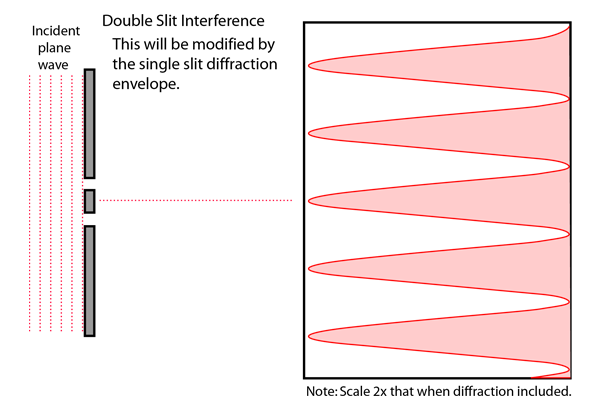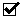Double slit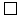Three slitsFour slitsFive slitsDiffraction and interferenceInterference only

Under the Fraunhofer conditions, the light curve of a multiple slit arrangement will be the interference pattern multiplied by the single slit diffraction envelope. This assumes that all the slits are identical.

 Show intensity comparison
Index

Diffraction concepts

Fraunhofer diffraction

Interference concepts

 HyperPhysics***** Light and Vision R Nave
Go Back

# Three Slit Interference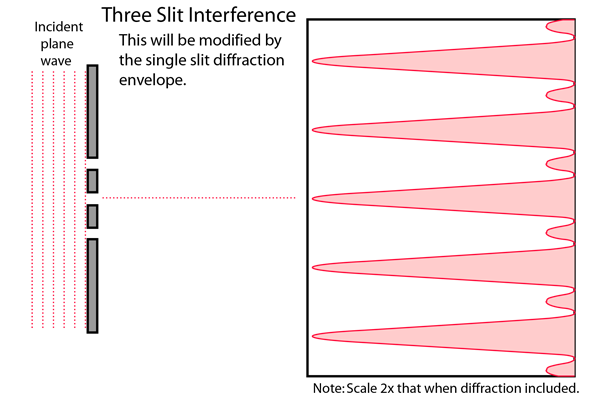Double slitThree slitsFour slitsFive slitsDiffraction and interferenceInterference only

Under the Fraunhofer conditions, the light curve of a multiple slit arrangement will be the interference pattern multiplied by the single slit diffraction envelope. This assumes that all the slits are identical.

 Show intensity comparison
Index

Diffraction concepts

Fraunhofer diffraction

Interference concepts

 HyperPhysics***** Light and Vision R Nave
Go Back

# Four Slit Interference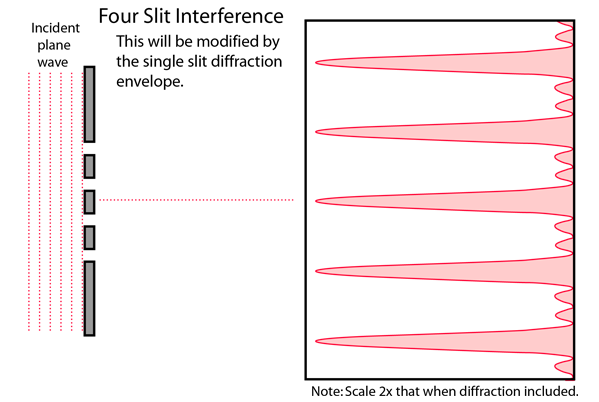Double slitThree slitsFour slitsFive slitsInterference only

Under the Fraunhofer conditions, the light curve of a multiple slit arrangement will be the interference pattern multiplied by the single slit diffraction envelope. This assumes that all the slits are identical.

 Show intensity comparison
Index

Diffraction concepts

Fraunhofer diffraction

Interference concepts

 HyperPhysics***** Light and Vision R Nave
Go Back

# Five Slit Interference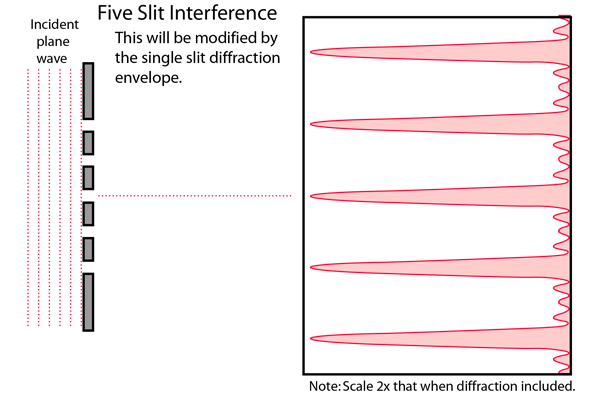Double slitThree slitsFour slitsFive slitsDiffraction and interferenceInterference only

Under the Fraunhofer conditions, the light curve of a multiple slit arrangement will be the interference pattern multiplied by the single slit diffraction envelope. This assumes that all the slits are identical.

 Show intensity comparison
Index

Diffraction concepts

Fraunhofer diffraction

Interference concepts

 HyperPhysics***** Light and Vision R Nave
Go Back